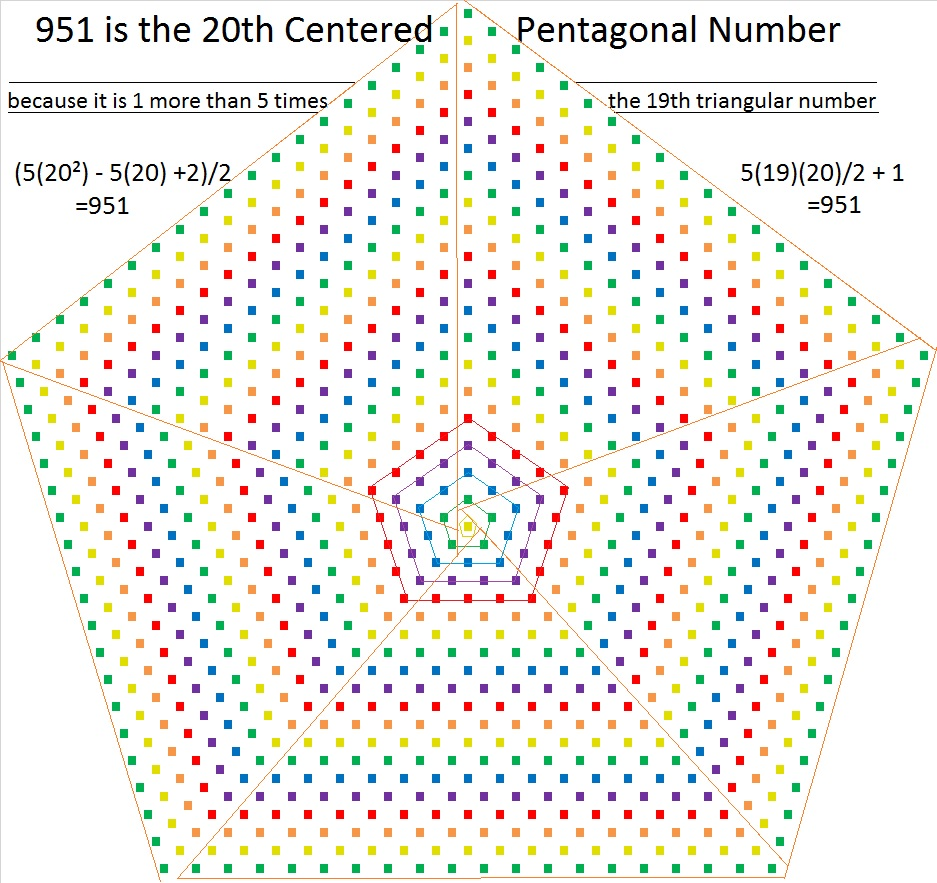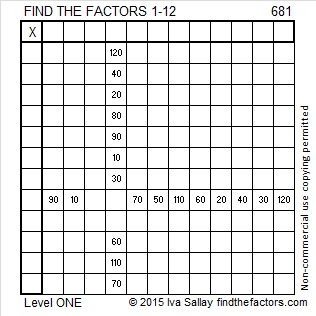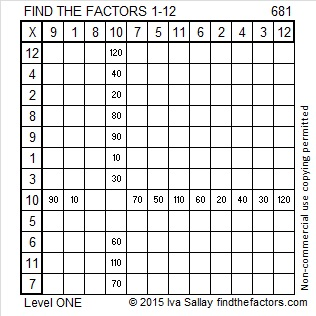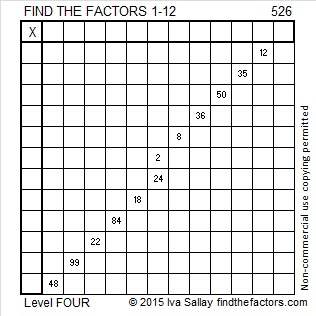# 951 is the 20th Centered Pentagonal Number

Since 951 is the 20th centered pentagonal number, I decided to make the following graphic with 20 concentric pentagons. I’ve outlined the pentagons in the center to make them clearer. The graphic also shows that 951 is one more than five times the 19th triangular number.951 is also the hypotenuse of a Pythagorean triple:
225-924-951 which is 3 times (75-308-317)

As numbers get bigger, palindromes in base 2 get rarer, but 951 is one of them:
1110110111 in BASE 2 because 1(2⁹) + 1(2⁸) + 1(2⁷) + 0(2⁶) + 1(2⁵) + 1(2⁴) + 0(2³) + 1(2²) + 1(2¹) +1(2⁰) = 951
It is also 1D1 in BASE 25 (D is 14 base 10) because 1(25²) + 13(25¹) + 1(25⁰) = 951

• 951 is a composite number.
• Prime factorization: 951 = 3 × 317
• The exponents in the prime factorization are 1 and 1. Adding one to each and multiplying we get (1 + 1)(1 + 1) = 2 × 2 = 4. Therefore 951 has exactly 4 factors.
• Factors of 951: 1, 3, 317, 951
• Factor pairs: 951 = 1 × 951 or 3 × 317
• 951 has no square factors that allow its square root to be simplified. √951 ≈ 30.83828789# 681 is a factor of the first 681 composite numbers added together

681 can be written as 0681, a number made with three consecutive numbers, 6, 8, and 10 so 681 and every other permutation of 6810 is divisible by 3. The number in the middle of those consecutive numbers is not divisible by 3, so none of those permutations are divisible by 9.

OEIS.org informs us that 681 is a factor of the first 681 composite numbers added together. Here are the number facts and the thought process I used to verify that claim:

• 1 is not a prime number, and it also is not a composite number.
• There are 123 prime numbers less than 681.
• 1 + 123 + 681 = 805, but there are 16 prime numbers between 681 and 805.
• 805 + 16 = 821, but there are 3 prime numbers from 805 to 821.
• 821 + 3 = 824, but there is 1 additional prime number between 821 and 824.
• 824 + 1 = 825 making it the 681st composite number.
• The sum of the numbers from 1 to 825 = (825)(826)/2 = 340,725.
• The sum of all the prime numbers from 2 to 823 is 53,342. (I listed all those prime numbers in excel and summed them.)
• The sum of the first 681 composite numbers is 340,725 – 53,342 – 1 = 287,382.
• (287,382)/681 is exactly 422. Thus 681 is a factor of the first 681 composite numbers added together.

[5(17²) + 5(17) + 2]/2 = 681 making 681 the 17th centered pentagonal number.

Here is a much less mind-boggling factoring puzzle:Print the puzzles or type the solution on this excel file: 12 Factors 2015-11-16

—————————————————————————————————

• 681 is a composite number.
• Prime factorization: 681 = 3 x 227
• The exponents in the prime factorization are 1 and 1. Adding one to each and multiplying we get (1 + 1)(1 + 1) = 2 x 2 = 4. Therefore 681 has exactly 4 factors.
• Factors of 681: 1, 3, 227, 681
• Factor pairs: 681 = 1 x 681 or 3 x 227
• 681 has no square factors that allow its square root to be simplified. √681 ≈ 26.0959767.—————————————————————————————————# 601 The Other Side of a Twin Prime

601 and 599 are twin primes because there is only one number between them. That number happens to be 600, a number with 24 factors.

Twin primes certainly are not identical twins. That solitary number between them and the fact that they are both primes is usually all they have in common.

Other famous twin primes are 11 and 13, 17 and 19, 29 and 31, and 41 and 43.

3, 5, 7 is the only prime triplet that consists of three consecutive odd numbers because 3 is the only prime number divisible by 3, and all sets of three consecutive odd numbers must have at least one number in the set that is divisible by 3. Other than 2, 3, 5, all other so-called prime triplets come from four consecutive odd numbers. Three of the four numbers will be prime while either the second or the third number will not be.

Twin primes like 599 and 601 can be prime factors. That is something 600 can never do.

For example, 599 x 601 = 359, 999 which is 600² – 1. They are its prime factors. 600 is a factor of an infinite amount of numbers, but it is not a prime factor of any number.

What are some ways twin primes are different from each other? I’ll use twin primes 599 and 601 as examples.

When each prime in a twin prime is divided by 4, the remainder will be 1 for one of them and 3 for the other. The one that has a remainder of 1 will be the sum of two square numbers and will also be the hypotenuse of a primitive Pythagorean triple. The one with a remainder of 3 will never be either of those things. In the triple and twin primes listed above 5, 13, 17, 29, 41, and 601 have a remainder of 1 when divided by 4.

601 = 24² + 5², and is the hypotenuse of the primitive Pythagorean triple 480, 551, 601 while 599 doesn’t do either of those things.

599 is the smallest number whose digits add up to 23. If you add up 601’s digits, you’ll get 7. Figure out how many numbers less than 601 have digits that add up to 7. It’s a lot.

601 has accomplished some other impressive things:1 + 5 + 10 + 15 + 20 + 25 + 30 + 35 + 40 + 45 + 50 + 55 + 60 + 65 + 70 + 75 = 601 which makes 601 the 16th centered pentagonal number

Because it is a centered pentagonal number, 601 is one more than five times the 15th triangular number.

The factors of numbers from 601 to 607 form a symmetrical shape.I gave the factoring information for 599 previously. Here is the information for 601:

• 601 is a prime number.
• Prime factorization: 601 is prime.
• The exponent of prime number 601 is 1. Adding 1 to that exponent we get (1 + 1) = 2. Therefore 601 has exactly 2 factors.
• Factors of 601: 1, 601
• Factor pairs: 601 = 1 x 601
• 601 has no square factors that allow its square root to be simplified. √601 ≈ 24.5153How do we know that 601 is a prime number? If 601 were not a prime number, then it would be divisible by at least one prime number less than or equal to √601 ≈ 24.5. Since 601 cannot be divided evenly by 2, 3, 5, 7, 11, 13, 17, 19, or 23, we know that 601 is a prime number.

# 526 is a Centered Pentagonal Number

I am excited that Kerrydunton included the Find the Factors puzzles on a list of great maths starters.

1 + 5 + 10 + 15 + 20 + 25 + 30 + 35 + 40 + 45 + 50 + 55 + 60 + 65 + 70 = 526. Thus, 526 is the 15th centered pentagonal number.

The above equation is the same as saying that 526 is one more than five times the 14th triangular number.The last digit of a centered pentagonal number is always 1 or 6. Triangular numbers greater than 3 are always composite numbers, but centered pentagonal numbers ending in 1 might be prime numbers. For example 31, 181, 331, 601, 1051, 1381, and 3331 are centered pentagonal numbers and prime numbers.Print the puzzles or type the solution on this excel file: 12 Factors 2015-06-15

—————————————————————————————————

• 526 is a composite number.
• Prime factorization: 526 = 2 x 263
• The exponents in the prime factorization are 1 and 1. Adding one to each and multiplying we get (1 + 1)(1 + 1) = 2 x 2 = 4. Therefore 526 has exactly 4 factors.
• Factors of 526: 1, 2, 263, 526
• Factor pairs: 526 = 1 x 526 or 2 x 263
• 526 has no square factors that allow its square root to be simplified. √526 ≈ 22.93468988—————————————————————————————————